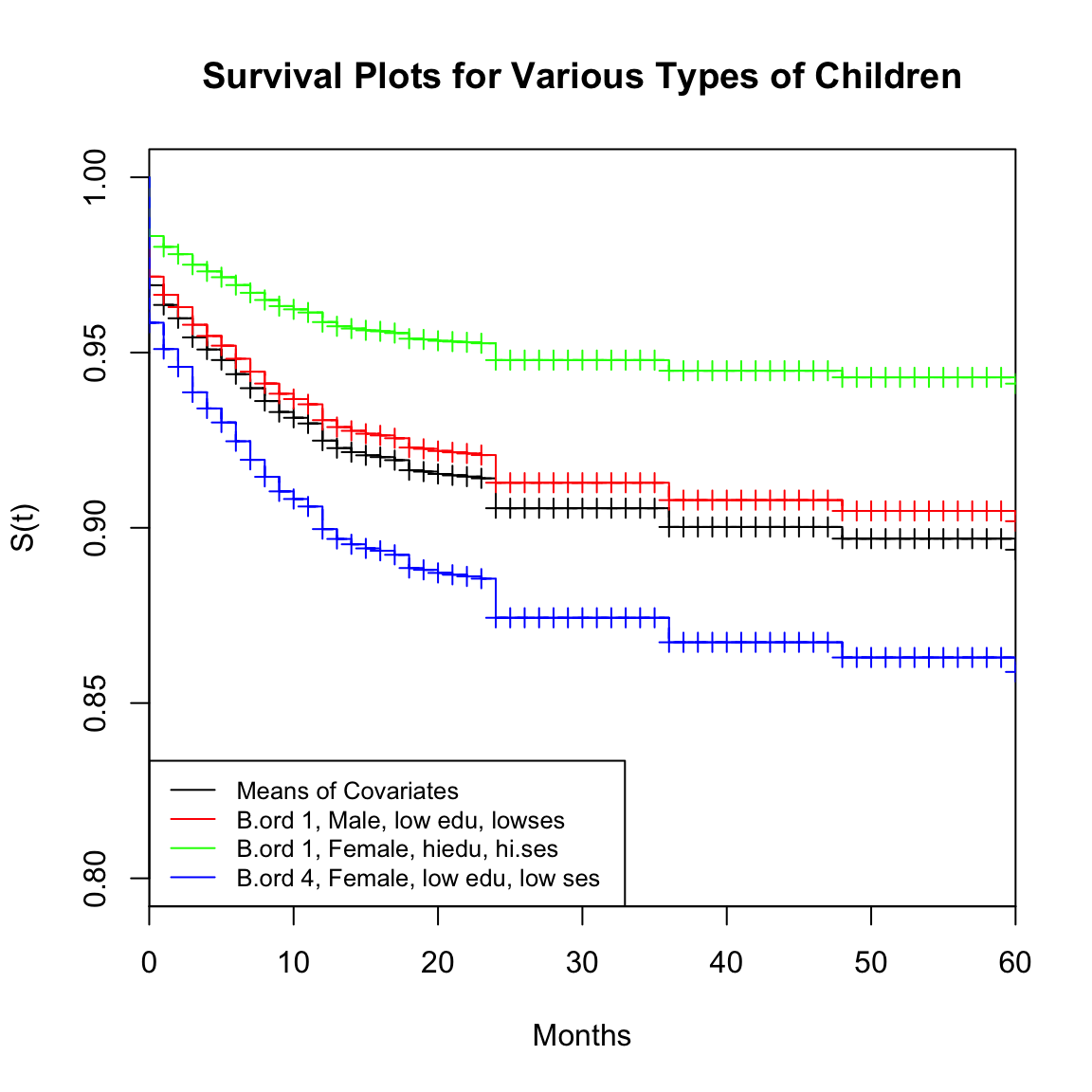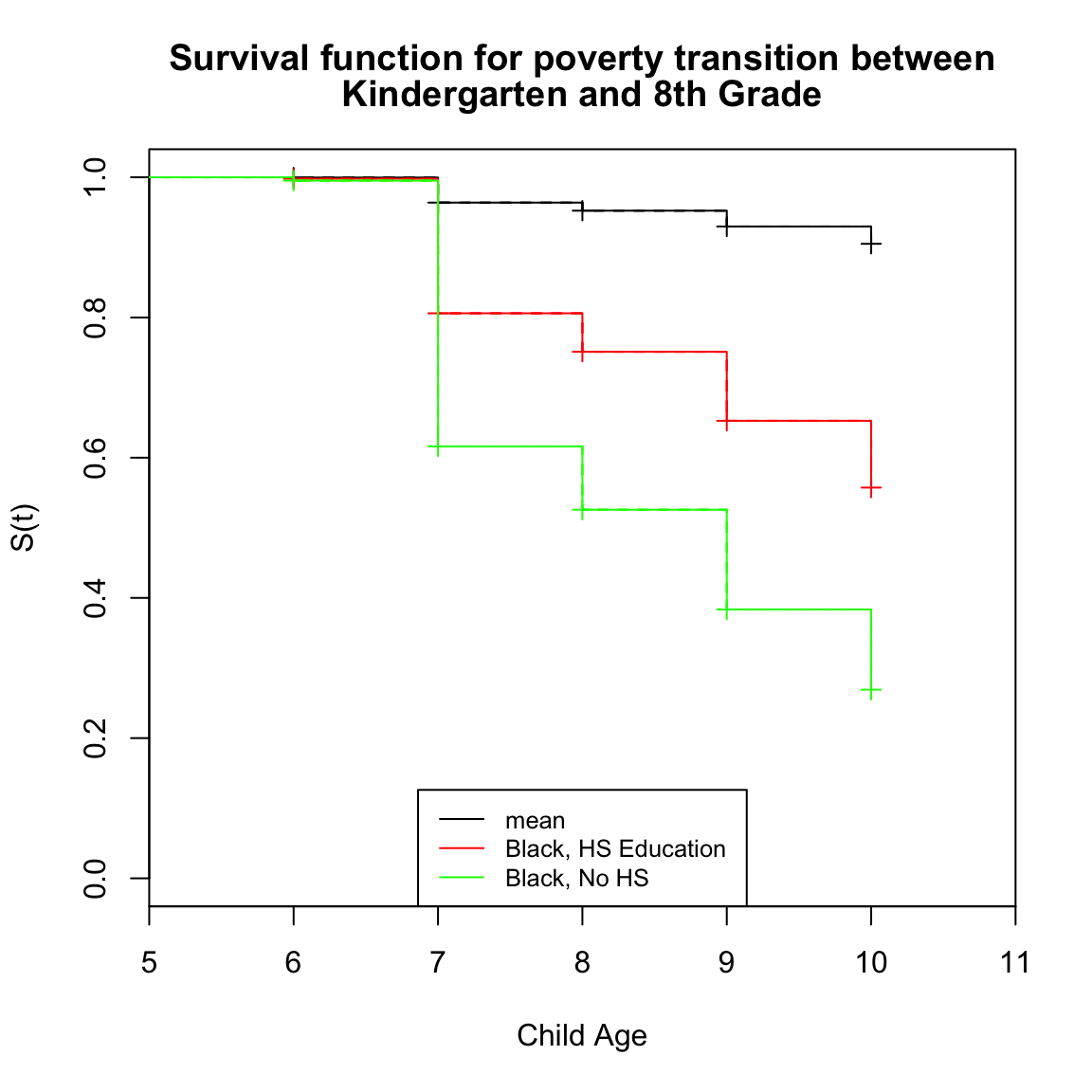This example will illustrate how to fit parametric the Cox Proportional hazards model to continuous duration data (i.e. person-level data) and a discrete-time (longitudinal) data set. In this example, I will use the event of a child dying before age 5 in Haiti. The data for this example come from the Haitian Demographic and Health Survey for 2012 birth recode file. This file contains information for all live births to women sampled in the survey.

The longitudinal data example uses data from the ECLS-K. Specifically, we will examine the transition into poverty between kindergarten and third grade.

#Load required libraries
library(foreign)
library(survival)
## Loading required package: splines
library(car)
library(survey)
## Loading required package: grid
##
## Attaching package: 'survey'
##
## The following object is masked from 'package:graphics':
##
##     dotchart
library(muhaz)

haiti<-read.dta("/Users/ozd504/Google Drive/dem7223/data/HTBR61FL.DTA", convert.factors = F)
#We form a subset of variables
sub<-data.frame(CASEID=haiti$caseid,v008=haiti$v008,bord=haiti$bidx,csex=haiti$b4,b2=haiti$b2, b3=haiti$b3, b5=haiti$b5, b7=haiti$b7, ibint=haiti$b11, rural=haiti$v025, educ=haiti$v106,age=haiti$v012,partneredu=haiti$v701,partnerage=haiti$v730, hhses=haiti$v190, weight=haiti$v005/1000000, psu=haiti$v021, strata=haiti$v022)

sub$death.age<-ifelse(sub$b5==1,
((((sub$v008))+1900)-(((sub$b3))+1900))
,sub$b7) #censoring indicator for death by age 5, in months (<=60 months) sub$d.event<-ifelse(is.na(sub$b7)==T|sub$b7>60,0,1)
sub$d.eventfac<-factor(sub$d.event); levels(sub$d.eventfac)<-c("Alive at Age 5", "Dead by Age 5") table(sub$d.eventfac)
##
## Alive at Age 5  Dead by Age 5
##          25981           3032
#recodes
sub$male<-ifelse(sub$csex==1,1,0)
sub$educ.high<-ifelse(sub$educ %in% c(2,3), 1, 0)
sub$age2<-sub$age^2
sub$partnerhiedu<-ifelse(sub$partneredu<3,0,ifelse(sub$partneredu%in%c(8,9),NA,1 )) ### Fit the model #using coxph in survival library fit.cox2<-coxph(Surv(death.age, d.event)~I(bord>2)+male+educ.high+I(age/5)+I(hhses>3), data=sub) summary(fit.cox2) ## Call: ## coxph(formula = Surv(death.age, d.event) ~ I(bord > 2) + male + ## educ.high + I(age/5) + I(hhses > 3), data = sub) ## ## n= 29013, number of events= 3032 ## ## coef exp(coef) se(coef) z Pr(>|z|) ## I(bord > 2)TRUE 0.51264 1.66969 0.04080 12.57 < 2e-16 *** ## male 0.15091 1.16289 0.03647 4.14 3.5e-05 *** ## educ.high -0.27103 0.76260 0.05911 -4.59 4.5e-06 *** ## I(age/5) 0.00408 1.00409 0.01264 0.32 0.747 ## I(hhses > 3)TRUE -0.11566 0.89078 0.04744 -2.44 0.015 * ## --- ## Signif. codes: 0 '***' 0.001 '**' 0.01 '*' 0.05 '.' 0.1 ' ' 1 ## ## exp(coef) exp(-coef) lower .95 upper .95 ## I(bord > 2)TRUE 1.670 0.599 1.541 1.809 ## male 1.163 0.860 1.083 1.249 ## educ.high 0.763 1.311 0.679 0.856 ## I(age/5) 1.004 0.996 0.980 1.029 ## I(hhses > 3)TRUE 0.891 1.123 0.812 0.978 ## ## Concordance= 0.588 (se = 0.005 ) ## Rsquare= 0.01 (max possible= 0.881 ) ## Likelihood ratio test= 300 on 5 df, p=0 ## Wald test = 283 on 5 df, p=0 ## Score (logrank) test = 290 on 5 df, p=0 plot(survfit(fit.cox2), ylim=c(.8,1), xlim=c(0,60), ylab="S(t)", xlab="Age in Months") title(main="Survival Function for Child Mortality")#Now I will do some basic model building, I will do a LRT for nested models #this is much easier using th coxph function in the survival library fit.cox1<-coxph(Surv(death.age, d.event)~I(bord>2)+male, data=sub) #LRT anova(fit.cox2, fit.cox1) ## Analysis of Deviance Table ## Cox model: response is Surv(death.age, d.event) ## Model 1: ~ I(bord > 2) + male + educ.high + I(age/5) + I(hhses > 3) ## Model 2: ~ I(bord > 2) + male ## loglik Chisq Df P(>|Chi|) ## 1 -30687 ## 2 -30708 42 3 4e-09 *** ## --- ## Signif. codes: 0 '***' 0.001 '**' 0.01 '*' 0.05 '.' 0.1 ' ' 1 #AIC extractAIC(fit.cox1) ##  2 61420 extractAIC(fit.cox2) ##  5 61384 #use survey design des<-svydesign(ids=~psu, strata = ~strata , weights=~weight, data=sub) cox.s<-svycoxph(Surv(death.age, d.event)~I(bord>2)+male+educ.high+I(age/5)+I(hhses>3), design=des) summary(cox.s) ## Stratified 1 - level Cluster Sampling design (with replacement) ## With (445) clusters. ## svydesign(ids = ~psu, strata = ~strata, weights = ~weight, data = sub) ## Call: ## svycoxph(formula = Surv(death.age, d.event) ~ I(bord > 2) + male + ## educ.high + I(age/5) + I(hhses > 3), design = des) ## ## n= 29013, number of events= 3032 ## ## coef exp(coef) se(coef) z Pr(>|z|) ## I(bord > 2)TRUE 0.551513 1.735878 0.053495 10.31 < 2e-16 *** ## male 0.164216 1.178469 0.041113 3.99 6.5e-05 *** ## educ.high -0.300899 0.740153 0.082459 -3.65 0.00026 *** ## I(age/5) -0.000583 0.999417 0.019245 -0.03 0.97583 ## I(hhses > 3)TRUE -0.066679 0.935495 0.063104 -1.06 0.29067 ## --- ## Signif. codes: 0 '***' 0.001 '**' 0.01 '*' 0.05 '.' 0.1 ' ' 1 ## ## exp(coef) exp(-coef) lower .95 upper .95 ## I(bord > 2)TRUE 1.736 0.576 1.563 1.93 ## male 1.178 0.849 1.087 1.28 ## educ.high 0.740 1.351 0.630 0.87 ## I(age/5) 0.999 1.001 0.962 1.04 ## I(hhses > 3)TRUE 0.935 1.069 0.827 1.06 ## ## Concordance= 0.591 (se = 0.005 ) ## Rsquare= NA (max possible= NA ) ## Likelihood ratio test= NA on 5 df, p=NA ## Wald test = 205 on 5 df, p=0 ## Score (logrank) test = NA on 5 df, p=NA #plot some survival function estimates for various types of children plot(survfit(cox.s, conf.int = F), ylim=c(.8,1), xlim=c(0,60), ylab="S(t)", xlab="Months") title (main = "Survival Plots for Various Types of Children") lines(survfit(cox.s, newdata=data.frame(bord=1,male =1,educ.high=0,age=30,hhses=1), conf.int = F), col="red") lines(survfit(cox.s, newdata=data.frame(bord=1,male =0,educ.high=1,age=30,hhses=5), conf.int = F), col="green") lines(survfit(cox.s, newdata=data.frame(bord=4,male =0,educ.high=0,age=30,hhses=1), conf.int = F), col="blue") legend("bottomleft", legend=c("Means of Covariates", "B.ord 1, Male, low edu, lowses", "B.ord 1, Female, hiedu, hi.ses", "B.ord 4, Female, low edu, low ses"), lty=1, col=c(1,"red", "green", "blue"), cex=.8)# Using Longitudinal Data As in the other examples, I illustrate fitting these models to data that are longitudinal, instead of person-duration. In this example, we will examine how to fit the Cox model to a longitudinally collected data set. First we load our data load("~/Google Drive/dem7903_App_Hier/data/eclsk.Rdata") names(eclsk)<-tolower(names(eclsk)) #get out only the variables I'm going to use for this example myvars<-c( "childid","gender", "race", "r1_kage","r4age", "r5age", "r6age", "r7age","c1r4mtsc", "c4r4mtsc", "c5r4mtsc", "c6r4mtsc", "c7r4mtsc", "w1povrty","w1povrty","w3povrty", "w5povrty", "w8povrty","wkmomed", "s2_id", "c1_5fp0", "c15fpstr", "c15fppsu") eclsk<-eclsk[,myvars] eclsk$age1<-ifelse(eclsk$r1_kage==-9, NA, eclsk$r1_kage/12)
eclsk$age2<-ifelse(eclsk$r4age==-9, NA, eclsk$r4age/12) #for the later waves, the NCES group the ages into ranges of months, so 1= <105 months, 2=105 to 108 months. So, I fix the age at the midpoint of the interval they give, and make it into years by dividing by 12 eclsk$age3<-recode(eclsk$r5age,recodes="1=105; 2=107; 3=109; 4=112; 5=115; 6=117; -9=NA")/12 eclsk$pov1<-ifelse(eclsk$w1povrty==1,1,0) eclsk$pov2<-ifelse(eclsk$w3povrty==1,1,0) eclsk$pov3<-ifelse(eclsk$w5povrty==1,1,0) #Recode race with white, non Hispanic as reference using dummy vars eclsk$hisp<-recode (eclsk$race, recodes="3:4=1;-9=NA; else=0") eclsk$black<-recode (eclsk$race, recodes="2=1;-9=NA; else=0") eclsk$asian<-recode (eclsk$race, recodes="5=1;-9=NA; else=0") eclsk$nahn<-recode (eclsk$race, recodes="6:7=1;-9=NA; else=0") eclsk$other<-recode (eclsk$race, recodes="8=1;-9=NA; else=0") eclsk$male<-recode(eclsk$gender, recodes="1=1; 2=0; -9=NA") eclsk$mlths<-recode(eclsk$wkmomed, recodes = "1:2=1; 3:9=0; else = NA") eclsk$mgths<-recode(eclsk$wkmomed, recodes = "1:3=0; 4:9=1; else =NA")  Now, I need to form the transition variable, this is my event variable, and in this case it will be 1 if a child enters poverty between the first wave of the data and the third grade wave, and 0 otherwise. NOTE I need to remove any children who are already in poverty age wave 1, because they are not at risk of experiencing this particular transition. eclsk<-subset(eclsk, is.na(pov1)==F&is.na(pov2)==F&is.na(pov3)==F&is.na(age1)==F&is.na(age2)==F&is.na(age3)==F&pov1!=1&is.na(eclsk$c15fpstr)==F)
eclsk$povtran1<-ifelse(eclsk$pov1==0&eclsk$pov2==0, 0,1) eclsk$povtran2<-ifelse(eclsk$povtran1==1, NA,ifelse(eclsk$pov2==0&eclsk$pov3==0,0,1)) Now we do the entire data set. To analyze data longitudinally, we need to reshape the data from the current “wide” format (repeated measures in columns) to a “long” format (repeated observations in rows). The reshape() function allows us to do this easily. It allows us to specify our repeated measures, time varying covariates as well as time-constant covariates. e.long<-reshape(eclsk, idvar="childid", varying=list(age=c("age1","age2"), age2=c("age2", "age3"), povtran=c("povtran1", "povtran2")), times=1:2, direction="long" , drop = names(eclsk)[4:20]) e.long<-e.long[order(e.long$childid, e.long$time),] #find which kids failed in the first time period and remove them from the second risk period risk set failed1<-which(is.na(e.long$povtran1)==T)
e.long<-e.long[-failed1,]
e.long$age1r<-round(e.long$age1, 0)
e.long$age2r<-round(e.long$age2, 0)
head(e.long, n=10)
##             childid gender race c1_5fp0 c15fpstr c15fppsu pov1 pov2 pov3
## 0001002C.1 0001002C      2    1   352.4       63        1    0    0    0
## 0001002C.2 0001002C      2    1   352.4       63        1    0    0    0
## 0001007C.1 0001007C      1    1   359.0       63        1    0    0    0
## 0001007C.2 0001007C      1    1   359.0       63        1    0    0    0
## 0001010C.1 0001010C      2    1   352.4       63        1    0    0    0
## 0001010C.2 0001010C      2    1   352.4       63        1    0    0    0
## 0002004C.1 0002004C      2    1   228.1       63        1    0    0    0
## 0002004C.2 0002004C      2    1   228.1       63        1    0    0    0
## 0002006C.1 0002006C      2    1   287.2       63        1    0    1    1
## 0002008C.1 0002008C      2    1   259.2       63        1    0    0    0
##            hisp black asian nahn other male mlths mgths time  age1  age2
## 0001002C.1    0     0     0    0     0    0     0     0    1 6.433 7.894
## 0001002C.2    0     0     0    0     0    0     0     0    2 7.894 9.750
## 0001007C.1    0     0     0    0     0    1     0     1    1 5.300 6.825
## 0001007C.2    0     0     0    0     0    1     0     1    2 6.825 8.917
## 0001010C.1    0     0     0    0     0    0     0     1    1 5.886 7.386
## 0001010C.2    0     0     0    0     0    0     0     1    2 7.386 9.333
## 0002004C.1    0     0     0    0     0    0     0     1    1 5.450 6.978
## 0002004C.2    0     0     0    0     0    0     0     1    2 6.978 9.083
## 0002006C.1    0     0     0    0     0    0    NA    NA    1 5.158 6.686
## 0002008C.1    0     0     0    0     0    0     0     1    1 5.906 7.433
##            povtran1 age1r age2r
## 0001002C.1        0     6     8
## 0001002C.2        0     8    10
## 0001007C.1        0     5     7
## 0001007C.2        0     7     9
## 0001010C.1        0     6     7
## 0001010C.2        0     7     9
## 0002004C.1        0     5     7
## 0002004C.2        0     7     9
## 0002006C.1        1     5     7
## 0002008C.1        0     6     7

Now we fit the Cox model using full survey design. In the ECLS-K, I use the longitudinal weight for waves 1-5, as well as the associated psu and strata id’s for the longitudinal data from these waves from the parents of the child, since no data from the child themselves are used in the outcome.

des2<-svydesign(ids = ~c15fppsu, strata = ~c15fpstr, weights=~c1_5fp0, data=e.long, nest=T)

#Fit the model
fitl1<-svycoxph(Surv(time = age1r, time2 = age2r, event = povtran1)~mlths+mgths+black+hisp+other+nahn, design=des2)
## Warning: Stop time must be > start time, NA created
## Warning: Stop time must be > start time, NA created
summary(fitl1) 
## Stratified 1 - level Cluster Sampling design (with replacement)
## With (501) clusters.
## svydesign(ids = ~c15fppsu, strata = ~c15fpstr, weights = ~c1_5fp0,
##     data = e.long, nest = T)
## Call:
## svycoxph(formula = Surv(time = age1r, time2 = age2r, event = povtran1) ~
##     mlths + mgths + black + hisp + other + nahn, design = des2)
##
##   n= 12978, number of events= 616
##    (159 observations deleted due to missingness)
##
##         coef exp(coef) se(coef)     z Pr(>|z|)
## mlths  0.809     2.246    0.125  6.45  1.1e-10 ***
## mgths -0.995     0.370    0.124 -7.99  1.3e-15 ***
## black  1.371     3.941    0.155  8.86  < 2e-16 ***
## hisp   0.837     2.310    0.114  7.37  1.7e-13 ***
## other  0.932     2.539    0.320  2.91   0.0036 **
## nahn   1.176     3.240    0.362  3.24   0.0012 **
## ---
## Signif. codes:  0 '***' 0.001 '**' 0.01 '*' 0.05 '.' 0.1 ' ' 1
##
##       exp(coef) exp(-coef) lower .95 upper .95
## mlths      2.25      0.445      1.76     2.871
## mgths      0.37      2.705      0.29     0.472
## black      3.94      0.254      2.91     5.337
## hisp       2.31      0.433      1.85     2.886
## other      2.54      0.394      1.36     4.755
## nahn       3.24      0.309      1.59     6.593
##
## Concordance= 0.751  (se = 0.01 )
## Rsquare= NA   (max possible= NA )
## Likelihood ratio test= NA  on 6 df,   p=NA
## Wald test            = 484  on 6 df,   p=0
## Score (logrank) test = NA  on 6 df,   p=NA
plot(survfit(fitl1, conf.int = F), ylab="S(t)", xlab="Child Age", xlim=c(5, 11))
## Warning: Stop time must be > start time, NA created
lines(survfit(fitl1, newdata = data.frame(mlths=0, mgths=0, black=1, hisp=0, other=0, nahn=0), conf.int=F) ,col="red")
## Warning: Stop time must be > start time, NA created
lines(survfit(fitl1, newdata = data.frame(mlths=1, mgths=0, black=1, hisp=0, other=0, nahn=0), conf.int=F) ,col="green")
## Warning: Stop time must be > start time, NA created
title(main=c("Survival function for poverty transition between","Kindergarten and 8th Grade"))
legend("bottom", legend=c("mean", "Black, HS Education", "Black, No HS"), lty=1, col=c(1,"red", "green"), cex=.8)